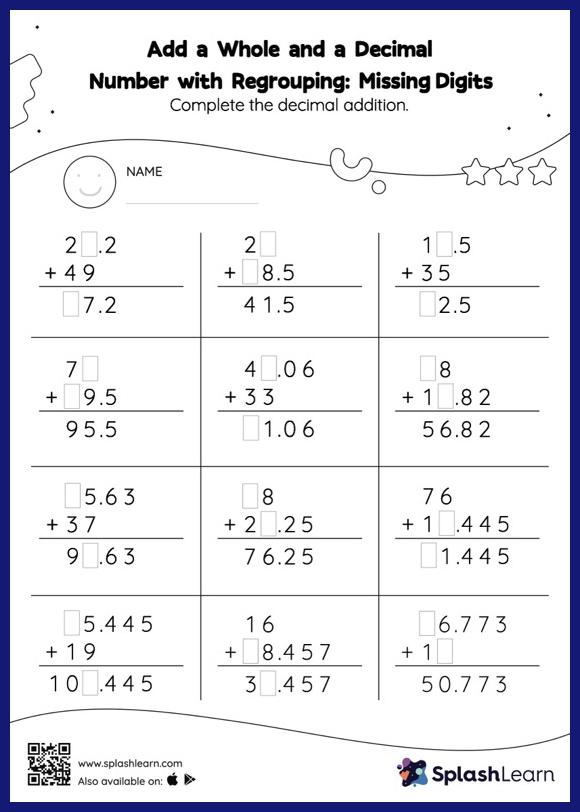# Add a Whole and a Decimal Number with Regrouping: Missing Digits Worksheet

Home > Add a Whole and a Decimal Number with Regrouping: Missing DigitsThis worksheet consists of a set of questions on addition where students are required to add a whole and a decimal number with regrouping. Students use the relationship between addition and subtraction to find the missing number while adding decimals. To reach the answer, they must also regroup the numbers in add a whole and a decimal number with regrouping worksheet using the relationships between ones, tenths, hundredths, thousandths, etc.